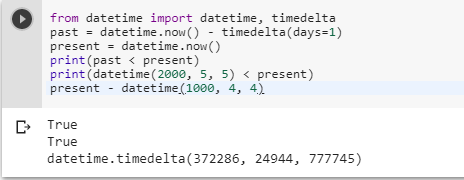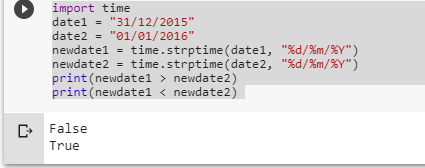2 views
in Python

How would I compare two dates to see which is later, using Python?

For example, I want to check if the current date is past the last date in this list I am creating, of holiday dates, so that it will send an email automatically, telling the admin to update the holiday.txt file

by (106k points)

There are many ways by which you can compare two dates some of the important methods are as follows:-

The first thing you can use the datetime module and the operator <. Below is an example that shows how we use the datetime module.

from datetime import datetime, timedelta

past = datetime.now() - timedelta(days=1)

present = datetime.now()

print(past < present)

print(datetime(2000, 5, 5) < present)

present - datetime(1000, 4, 4)Another thing we can use is the time module:-

Let’s assume two dates first:

date1 = "31/12/2015"

date2 = "01/01/2016"

You can do the following:

newdate1 = time.strptime(date1, "%d/%m/%Y")

newdate2 = time.strptime(date2, "%d/%m/%Y")

Now for converting them into python's date format. You need to do the comparison:

newdate1 > newdate2

newdate1 < newdate2

The full code of the above explanation:-

import time

date1 = "31/12/2015"

date2 = "01/01/2016"

newdate1 = time.strptime(date1, "%d/%m/%Y")

newdate2 = time.strptime(date2, "%d/%m/%Y")

print(newdate1 > newdate2)

print(newdate1 < newdate2)